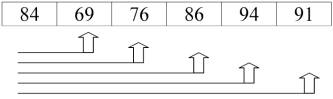Exchange SortThe exchange sort is similar to its cousin, the bubble sort, in that it compares elements of the array and swaps those that are not in their proper positions.  (Some people refer to the "exchange sort" as a "bubble sort".)  The difference between these two sorts is the manner in which they compare the elements. The exchange sort compares the first element with each following element of the array, making any necessary swaps.When the first pass through the array is complete, the exchange sort then takes the second element and compares it with each following element of the array swapping elements that are out of order.  This sorting process continues until the entire array is ordered.

Let's examine our same table of elements again using an exchange sort for descending order.  Remember, a "pass" is defined as one full trip through the array comparing and if necessary, swapping elements.

 Array at beginning: 84 69 76 86 94 91 After Pass #1: 94 69 76 84 86 91 After Pass #2: 94 91 69 76 84 86 After Pass #3: 94 91 86 69 76 84 After Pass #4: 94 91 86 84 69 76 After Pass #5 (done): 94 91 86 84 76 69

The exchange sort, in some situations, is slightly more efficient than the bubble sort.  It is not necessary for the exchange sort to make that final complete pass needed by the bubble sort to determine that it is finished.

//Exchange Sort Function for Descending Order
void ExchangeSort(apvector <int> &num)
{
int i, j;
int temp;   // holding variable
int numLength = num.length( );
for (i=0; i< (numLength -1); i++)    // element to be compared
{
for(j = (i+1); j < numLength; j++)   // rest of the elements
{
if (num[i] < num[j])          // descending order
{
temp= num[i];          // swap
num[i] = num[j];
num[j] = temp;
}
}
}
return;
}